# Convolutional Neural Network (CNN) Architectures

Convolutional Neural Network(CNN) is a neural network architecture in Deep Learning, used to recognize the pattern from structured arrays. However, over many years, CNN architectures have evolved. Many variants of the fundamental CNN Architecture This been developed, leading to amazing advances in the growing deep-learning field.

Let’s discuss, How CNN architecture developed and grow over time.

### 1. LeNet-5

• The First LeNet-5 architecture is the most widely known CNN architecture. It was introduced in 1998 and is widely used for handwritten method digit recognition.
•  LeNet-5 has 2 convolutional and 3 full layers.
• This LeNet-5 architecture has 60,000 parameters.LeNet-5

• The LeNet-5 has the ability to process higher one-resolution images that require larger and more CNN convolutional layers.
• The leNet-5 technique is measured by the availability of all computing resources

Example Model of LeNet-5

## Python3

 `import` `torch ` `from` `torchsummary ``import` `summary ` `import` `torch.nn as nn ` `import` `torch.nn.functional as F ` ` `  `class` `LeNet5(nn.Module): ` `    ``def` `__init__(``self``): ` `        ``# Call the parent class's init method ` `        ``super``(LeNet5, ``self``).__init__() ` `         `  `        ``# First Convolutional Layer ` `        ``self``.conv1 ``=` `nn.Conv2d(in_channels``=``1``, out_channels``=``6``, kernel_size``=``5``, stride``=``1``) ` `         `  `        ``# Max Pooling Layer ` `        ``self``.pool ``=` `nn.MaxPool2d(kernel_size``=``2``, stride``=``2``) ` `         `  `        ``# Second Convolutional Layer ` `        ``self``.conv2 ``=` `nn.Conv2d(in_channels``=``6``, out_channels``=``16``, kernel_size``=``5``, stride``=``1``) ` `         `  `        ``# First Fully Connected Layer ` `        ``self``.fc1 ``=` `nn.Linear(in_features``=``16` `*` `5` `*` `5``, out_features``=``120``) ` `         `  `        ``# Second Fully Connected Layer ` `        ``self``.fc2 ``=` `nn.Linear(in_features``=``120``, out_features``=``84``) ` `         `  `        ``# Output Layer ` `        ``self``.fc3 ``=` `nn.Linear(in_features``=``84``, out_features``=``10``) ` ` `  `    ``def` `forward(``self``, x): ` `        ``# Pass the input through the first convolutional layer and activation function ` `        ``x ``=` `self``.pool(F.relu(``self``.conv1(x))) ` `         `  `        ``# Pass the output of the first layer through  ` `        ``# the second convolutional layer and activation function ` `        ``x ``=` `self``.pool(F.relu(``self``.conv2(x))) ` `         `  `        ``# Reshape the output to be passed through the fully connected layers ` `        ``x ``=` `x.view(``-``1``, ``16` `*` `5` `*` `5``) ` `         `  `        ``# Pass the output through the first fully connected layer and activation function ` `        ``x ``=` `F.relu(``self``.fc1(x)) ` `         `  `        ``# Pass the output of the first fully connected layer through  ` `        ``# the second fully connected layer and activation function ` `        ``x ``=` `F.relu(``self``.fc2(x)) ` `         `  `        ``# Pass the output of the second fully connected layer through the output layer ` `        ``x ``=` `self``.fc3(x) ` `         `  `        ``# Return the final output ` `        ``return` `x ` `     `  `lenet5 ``=` `LeNet5() ` `print``(lenet5)`

Output:

```LeNet5(
(conv1): Conv2d(1, 6, kernel_size=(5, 5), stride=(1, 1))
(pool): MaxPool2d(kernel_size=2, stride=2, padding=0, dilation=1, ceil_mode=False)
(conv2): Conv2d(6, 16, kernel_size=(5, 5), stride=(1, 1))
(fc1): Linear(in_features=400, out_features=120, bias=True)
(fc2): Linear(in_features=120, out_features=84, bias=True)
(fc3): Linear(in_features=84, out_features=10, bias=True)
)```

#### Model Summary :

Print the summary of the lenet5  to check the params

## Python3

 `# add the cuda to the mode ` `device ``=` `torch.device(``"cuda"` `if` `torch.cuda.is_available() ``else` `"cpu"``) ` `lenet5.to(device) ` ` `  `#Print the summary of the model ` `summary(lenet5, (``1``, ``32``, ``32``))`

Output:

```----------------------------------------------------------------
Layer (type)               Output Shape         Param #
================================================================
Conv2d-1            [-1, 6, 28, 28]             156
MaxPool2d-2            [-1, 6, 14, 14]               0
Conv2d-3           [-1, 16, 10, 10]           2,416
MaxPool2d-4             [-1, 16, 5, 5]               0
Linear-5                  [-1, 120]          48,120
Linear-6                   [-1, 84]          10,164
Linear-7                   [-1, 10]             850
================================================================
Total params: 61,706
Trainable params: 61,706
Non-trainable params: 0
----------------------------------------------------------------
Input size (MB): 0.00
Forward/backward pass size (MB): 0.06
Params size (MB): 0.24
Estimated Total Size (MB): 0.30
----------------------------------------------------------------```

### 2. AlexNNet

• The AlexNet CNN architecture won the 2012 ImageNet ILSVRC challenges of deep learning algorithm by a large variance by achieving 17% with top-5 error rate as the second best achieved 26%!
• It was introduced by Alex Krizhevsky (name of founder), The Ilya Sutskever and Geoffrey Hinton are quite similar to LeNet-5, only much bigger and deeper and it was introduced first to stack convolutional layers directly on top of each other models, instead of stacking a pooling layer top of each on CN network convolutional layer.
• AlexNNet has 60 million parameters as AlexNet has total 8 layers, 5 convolutional and 3 fully connected layers.
• AlexNNet is first to execute (ReLUs) Rectified Linear Units as activation functions
• it was the first CNN architecture that uses GPU to improve the performance.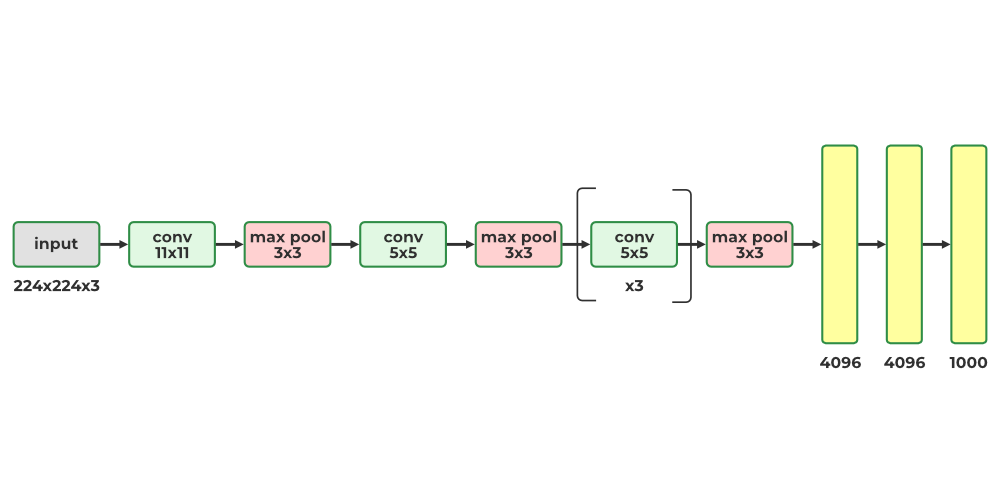ALexNNet

Example Model of AlexNNet

## Python3

 `import` `torch ` `from` `torchsummary ``import` `summary ` `import` `torch.nn as nn ` `import` `torch.nn.functional as F ` ` `  `class` `AlexNet(nn.Module): ` `    ``def` `__init__(``self``, num_classes``=``1000``): ` `        ``# Call the parent class's init method to initialize the base class ` `        ``super``(AlexNet, ``self``).__init__() ` `         `  `        ``# First Convolutional Layer with 11x11 filters, stride of 4, and 2 padding ` `        ``self``.conv1 ``=` `nn.Conv2d(in_channels``=``3``, out_channels``=``96``, kernel_size``=``11``, stride``=``4``, padding``=``2``) ` `         `  `        ``# Max Pooling Layer with a kernel size of 3 and stride of 2 ` `        ``self``.pool ``=` `nn.MaxPool2d(kernel_size``=``3``, stride``=``2``) ` `         `  `        ``# Second Convolutional Layer with 5x5 filters and 2 padding ` `        ``self``.conv2 ``=` `nn.Conv2d(in_channels``=``96``, out_channels``=``256``, kernel_size``=``5``, padding``=``2``) ` `         `  `        ``# Third Convolutional Layer with 3x3 filters and 1 padding ` `        ``self``.conv3 ``=` `nn.Conv2d(in_channels``=``256``, out_channels``=``384``, kernel_size``=``3``, padding``=``1``) ` `         `  `        ``# Fourth Convolutional Layer with 3x3 filters and 1 padding ` `        ``self``.conv4 ``=` `nn.Conv2d(in_channels``=``384``, out_channels``=``384``, kernel_size``=``3``, padding``=``1``) ` `         `  `        ``# Fifth Convolutional Layer with 3x3 filters and 1 padding ` `        ``self``.conv5 ``=` `nn.Conv2d(in_channels``=``384``, out_channels``=``256``, kernel_size``=``3``, padding``=``1``) ` `         `  `        ``# First Fully Connected Layer with 4096 output features ` `        ``self``.fc1 ``=` `nn.Linear(in_features``=``256` `*` `6` `*` `6``, out_features``=``4096``) ` `         `  `        ``# Second Fully Connected Layer with 4096 output features ` `        ``self``.fc2 ``=` `nn.Linear(in_features``=``4096``, out_features``=``4096``) ` `         `  `        ``# Output Layer with `num_classes` output features ` `        ``self``.fc3 ``=` `nn.Linear(in_features``=``4096``, out_features``=``num_classes) ` ` `  `    ``def` `forward(``self``, x): ` `        ``# Pass the input through the first convolutional layer and ReLU activation function ` `        ``x ``=` `self``.pool(F.relu(``self``.conv1(x))) ` `         `  `        ``# Pass the output of the first layer through  ` `        ``# the second convolutional layer and ReLU activation function ` `        ``x ``=` `self``.pool(F.relu(``self``.conv2(x))) ` `         `  `        ``# Pass the output of the second layer through  ` `        ``# the third convolutional layer and ReLU activation function ` `        ``x ``=` `F.relu(``self``.conv3(x)) ` `         `  `        ``# Pass the output of the third layer through  ` `        ``# the fourth convolutional layer and ReLU activation function ` `        ``x ``=` `F.relu(``self``.conv4(x)) ` `         `  `        ``# Pass the output of the fourth layer through  ` `        ``# the fifth convolutional layer and ReLU activation function ` `        ``x ``=` `self``.pool(F.relu(``self``.conv5(x))) ` `         `  `        ``# Reshape the output to be passed through the fully connected layers ` `        ``x ``=` `x.view(``-``1``, ``256` `*` `6` `*` `6``) ` `         `  `        ``# Pass the output through the first fully connected layer and activation function ` `        ``x ``=` `F.relu(``self``.fc1(x)) ` `        ``x ``=` `F.dropout(x, ``0.5``)     ` `         `  `        ``# Pass the output of the first fully connected layer through  ` `        ``# the second fully connected layer and activation function ` `        ``x ``=` `F.relu(``self``.fc2(x)) ` `         `  `        ``# Pass the output of the second fully connected layer through the output layer ` `        ``x ``=` `self``.fc3(x) ` `         `  `        ``# Return the final output ` `        ``return` `x ` `                                         `  `                                         `  `alexnet ``=` `AlexNet() ` `print``(alexnet)`

Output:

```AlexNet(
(conv1): Conv2d(3, 96, kernel_size=(11, 11), stride=(4, 4), padding=(2, 2))
(pool): MaxPool2d(kernel_size=3, stride=2, padding=0, dilation=1, ceil_mode=False)
(conv2): Conv2d(96, 256, kernel_size=(5, 5), stride=(1, 1), padding=(2, 2))
(conv3): Conv2d(256, 384, kernel_size=(3, 3), stride=(1, 1), padding=(1, 1))
(conv4): Conv2d(384, 384, kernel_size=(3, 3), stride=(1, 1), padding=(1, 1))
(conv5): Conv2d(384, 256, kernel_size=(3, 3), stride=(1, 1), padding=(1, 1))
(fc1): Linear(in_features=9216, out_features=4096, bias=True)
(fc2): Linear(in_features=4096, out_features=4096, bias=True)
(fc3): Linear(in_features=4096, out_features=1000, bias=True)
)```

#### Model Summary :

Print the summary of the alexnet to check the params

## Python3

 `# add the cuda to the mode ` `device ``=` `torch.device(``"cuda"` `if` `torch.cuda.is_available() ``else` `"cpu"``) ` `alexnet.to(device) ` ` `  `#Print the summary of the model ` `summary(alexnet, (``3``, ``224``, ``224``))`

Output:

```----------------------------------------------------------------
Layer (type)               Output Shape         Param #
================================================================
Conv2d-1           [-1, 96, 55, 55]          34,944
MaxPool2d-2           [-1, 96, 27, 27]               0
Conv2d-3          [-1, 256, 27, 27]         614,656
MaxPool2d-4          [-1, 256, 13, 13]               0
Conv2d-5          [-1, 384, 13, 13]         885,120
Conv2d-6          [-1, 384, 13, 13]       1,327,488
Conv2d-7          [-1, 256, 13, 13]         884,992
MaxPool2d-8            [-1, 256, 6, 6]               0
Linear-9                 [-1, 4096]      37,752,832
Linear-10                 [-1, 4096]      16,781,312
Linear-11                 [-1, 1000]       4,097,000
================================================================
Total params: 62,378,344
Trainable params: 62,378,344
Non-trainable params: 0
----------------------------------------------------------------
Input size (MB): 0.57
Forward/backward pass size (MB): 5.96
Params size (MB): 237.95
Estimated Total Size (MB): 244.49
----------------------------------------------------------------```

• The GoogleNet architecture was created by Christian Szegedy from Google Research and achieved a breakthrough result by lowering the top-5 error rate to below 7% in the ILSVRC 2014 challenge. This success was largely attributed to its deeper architecture than other CNNs, enabled by its inception modules which enabled more efficient use of parameters than preceding architectures
• GoogleNet has fewer parameters than AlexNet, with a ratio of 10:1 (roughly 6 million instead of 60 million)
• The architecture of the inception module looks as shown in Fig.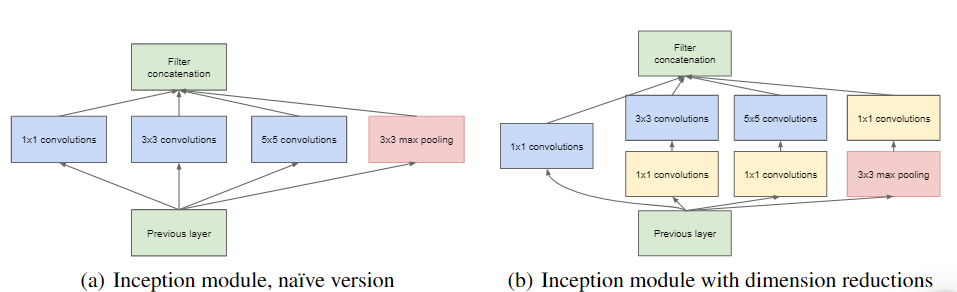• The notation “3 x 3 + 2(5)” means that the layer uses a 3 x 3 kernel, a stride of 2, and SAME padding. The input signal is then fed to four different layers, each with a RelU activation function and a stride of 1. These convolutional layers have varying kernel sizes (1 x 1, 3 x 3, and 5 x 5) to capture patterns at different scales. Additionally, each layer uses SAME padding, so all outputs have the same height and width as their inputs. This allows for the feature maps from all four top convolutional layers to be concatenated along the depth dimension in the final depth concat layer.
• The overall GoogleNet architecture has 22 larger deep CNN layers.

### 4. ResNet (Residual Network)

• Residual Network (ResNet), the winner of the ILSVRC 2015 challenge, was developed by Kaiming He and delivered an impressive top-5 error rate of 3.6% with an extremely deep CNN composed of 152 layers. An essential factor enabling the training of such a deep network is the use of skip connections (also known as shortcut connections). The signal that enters a layer is added to the output of a layer located higher up in the stack. Let’s explore why this is beneficial.
• When training a neural network, the goal is to make it replicate a target function h(x). By adding the input x to the output of the network (a skip connection), the network is made to model f(x) = h(x) – x, a technique known as residual learning.
`F(x) = H(x) - x which gives H(x) := F(x) + x. `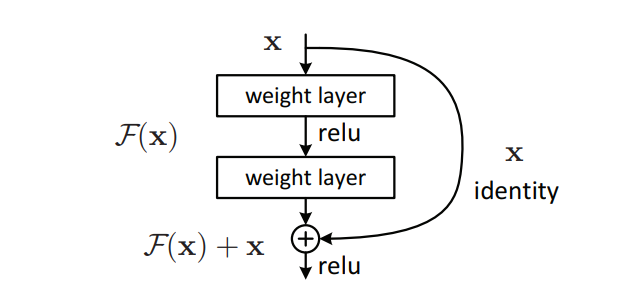Skip (Shortcut) connection

• When initializing a regular neural network, its weights are near zero, resulting in the network outputting values close to zero. With the addition of skip connections, the resulting network outputs a copy of its inputs, effectively modeling the identity function. This can be beneficial if the target function is similar to the identity function, as it will accelerate training. Furthermore, if multiple skip connections are added, the network can begin to make progress even if several layers have not yet begun learning.
• the target function is fairly close to the identity function (which is often the case), this will speed up training considerably. Moreover, if you add many skin connections, the network can start making progress even if several
• The deep residual network can be viewed as a series of residual units, each of which is a small neural network with a skip connection

### 5. DenseNet

• The DenseNet model introduced the concept of a densely connected convolutional network, where the output of each layer is connected to the input of every subsequent layer. This design principle was developed to address the issue of accuracy decline caused by the vanishing and exploding gradients in high-level neural networks.
• In simpler terms, due to the long distance between the input and output layer, the data is lost before it reaches its destination.
• The DenseNet model introduced the concept of a densely connected convolutional network, where the output of each layer is connected to the input of every subsequent layer. This design principle was developed to address the issue of accuracy decline caused by the vanishing and exploding gradients in high-level neural networks.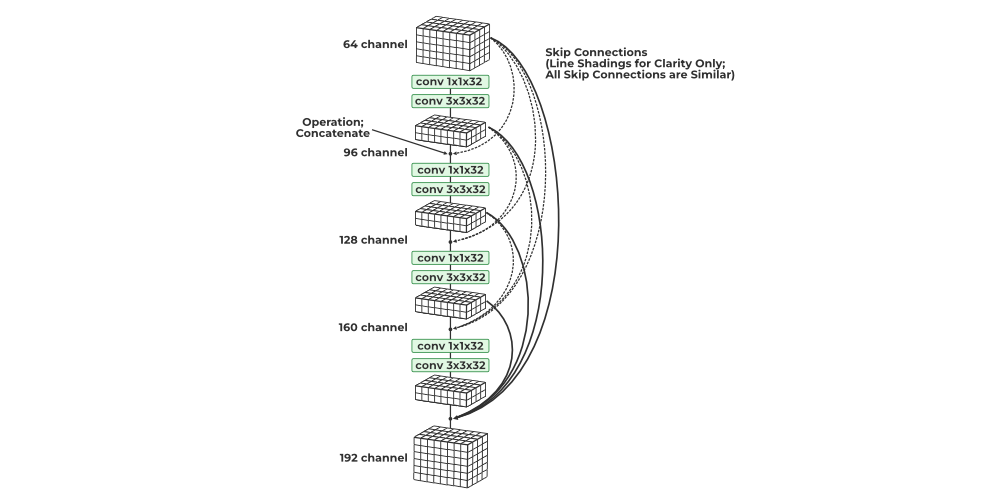DenseNet

• All convolutions in a dense block are ReLU-activated and use batch normalization. Channel-wise concatenation is only possible if the height and width dimensions of the data remain unchanged, so convolutions in a dense block are all of stride 1. Pooling layers are inserted between dense blocks for further dimensionality reduction.
• Intuitively, one might think that by concatenating all previously seen outputs, the number of channels and parameters would exponentially increase. However, DenseNet is surprisingly economical in terms of learnable parameters. This is because each concatenated block, which may have a relatively large number of channels, is first fed through a 1×1 convolution, reducing it to a small number of channels. Additionally, 1×1 convolutions are economical in terms of parameters. Then, a 3×3 convolution with the same number of channels is applied.
• The resulting channels from each step of the DenseNet are concatenated to the collection of all previously generated outputs. Each step, which utilizes a pair of 1×1 and 3×3 convolutions, adds K channels to the data. Consequently, the number of channels increases linearly with the number of convolutional steps in the dense block. The growth rate remains constant throughout the network, and DenseNet has demonstrated good performance with K values between 12 and 40.
• Dense blocks and pooling layers are combined to form a Tu DenseNet network. The DenseNet21 has 121 layers, however, the structure is adjustable and can readily be extended to more than 200 layers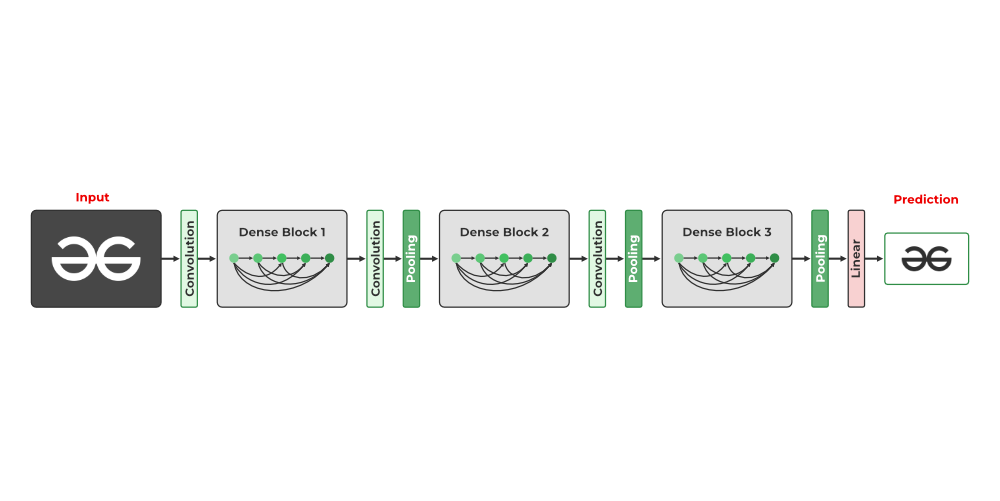DenseNet

Whether you're preparing for your first job interview or aiming to upskill in this ever-evolving tech landscape, GeeksforGeeks Courses are your key to success. We provide top-quality content at affordable prices, all geared towards accelerating your growth in a time-bound manner. Join the millions we've already empowered, and we're here to do the same for you. Don't miss out - check it out now!